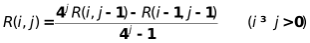matlab用辛普森公式求积分_数值计算实验9 数值积分实验

实验9数值积分实验

 成绩

T(0)=(b-a)(f(a)+f(b))/2 ; H(0) =(b-a)·f( (a+b)/2 ) ; T(i)=(T(i-1)+H(i-1))/2

T(i)的语义是将积分区间做2i 等分复化梯形求积分公式算出的近似值。

H(i)的语义是将积分区间做2i 等分时，将每个小区间的长度乘该小区间中点处函数值的乘积进行累加求和的结果。

R(i,0)=T(i)=(T(i-1)+H(i-1))/2, i>=0,T(i)为区间逐次减半递推梯形求积分公式算出的结果；

R(i,1)=S(i), i>=1,S(i)为由T(i)、T(i-1)联合递推得出的区间逐次减半递推辛普森求积分公式算出的结果；

R(i,2)=B(i), i>=2,B(i)为由S(i)、S(i-1)联合递推得出布尔求积分公式算出的结果；

,,,,,,,,,,;归纳总结有如下递推关系式1 要求上机实验前先编写出程序代码

2 编辑录入程序

3 调试程序并记录调试过程中出现的问题及修改程序的过程

4 经反复调试后，运行程序并验证程序运行是否正确。

5 记录运行时的输入和主要中间结果输出，以便与手工解算作比较。

6 要求在A4纸上手工计算并和计算机程序计算的主要中间结果进行比较，验证手工解算和机器解算的R(i, j)构成三角表是否一致。

function s = maromb(fun,a,b,tol)

%用龙贝格公式求积分

%格式：s = maromb(fun,a,b,tol)，fun 是被积函数，a,b是积分

%下、上限，tol 是容许误差，s 是返回积分近似值

if nargin < 4,tol = 1e-4;end

i = 1;j = 1;h = b - a;

T(1,1) = h * (feval(fun,a) + feval(fun,b)) / 2;

T(i+1,j) = T(i,j)/2 + sum(feval(fun,a + h/2:h:b - h/2)) * h/2;

T(i+1,j+1) = (4^j * T(i+1,j) - T(i,j)) / (4^j - 1);

while (abs(T(i+1,i+1) - T(i,i)) > tol)

i = i+1;

h = h/2;

T(i+1,1) = T(i,1)/2 + sum(feval(fun,a + h/2:h:b - h/2)) * h/2;

for j = 1:i

T(i+1,j+1) = (4^j * T(i+1,j) - T(i,j)) / (4^j - 1);

end

end

T

s = T(i+1,j+1);

>> fun = inline('x.^2');

>> a = 0;

>> b = 1;

>> tol = 1e-5;

>> s = maromb(fun,a,b,tol)

T =

0.5000         0         0

0.3750    0.3333         0

0.3438    0.3333    0.3333

s =

0.3333

通过本次实验我学会了使用龙贝格公式求积分的算法程序；掌握了使用龙贝格算法求解积分的本领；训练了我的编程的能力和把数学问题转化为算法的能力；在本次实验中我发现了自己在编程方面和思考问题方面的一些不足；在今后的学习中我的编程能力和编写算法的能力有待提高。

11-202394
11-281591
03-131万+
11-043万+
07-062万+
11-23
11-213034
03-23767
04-12242
03-18814
11-21502
11-20675
12-28
03-16239
09-112万+点击重新获取扫码支付余额充值